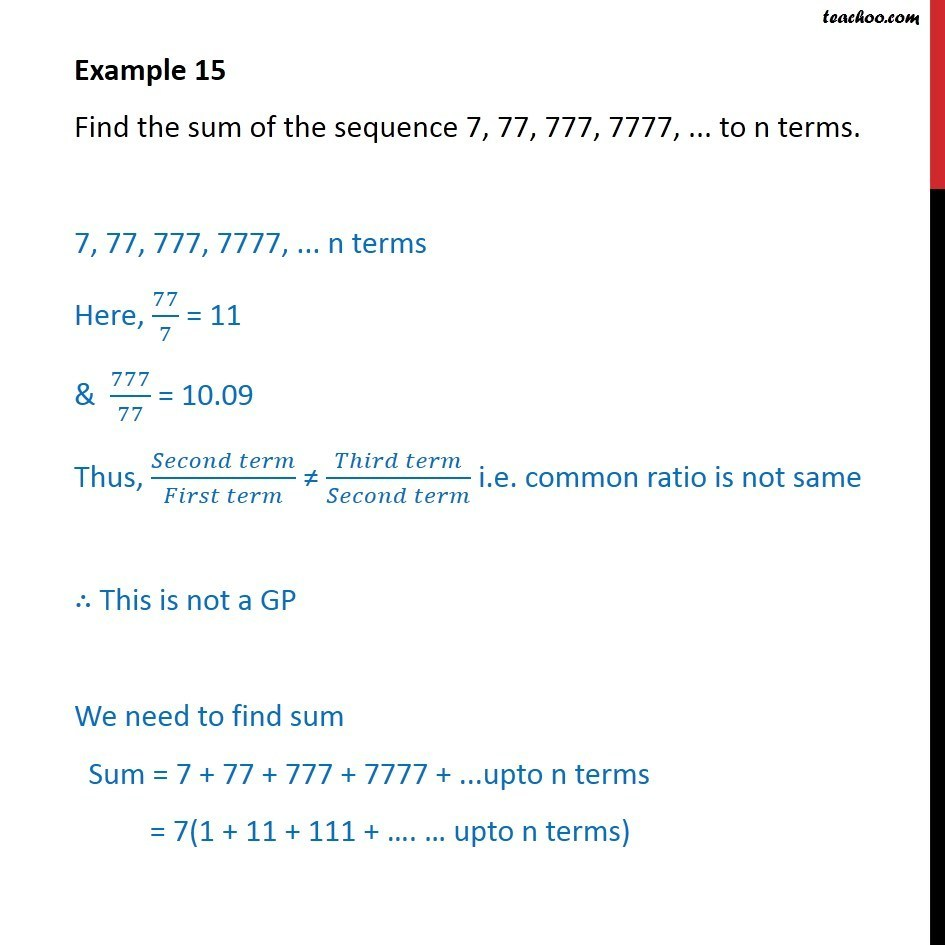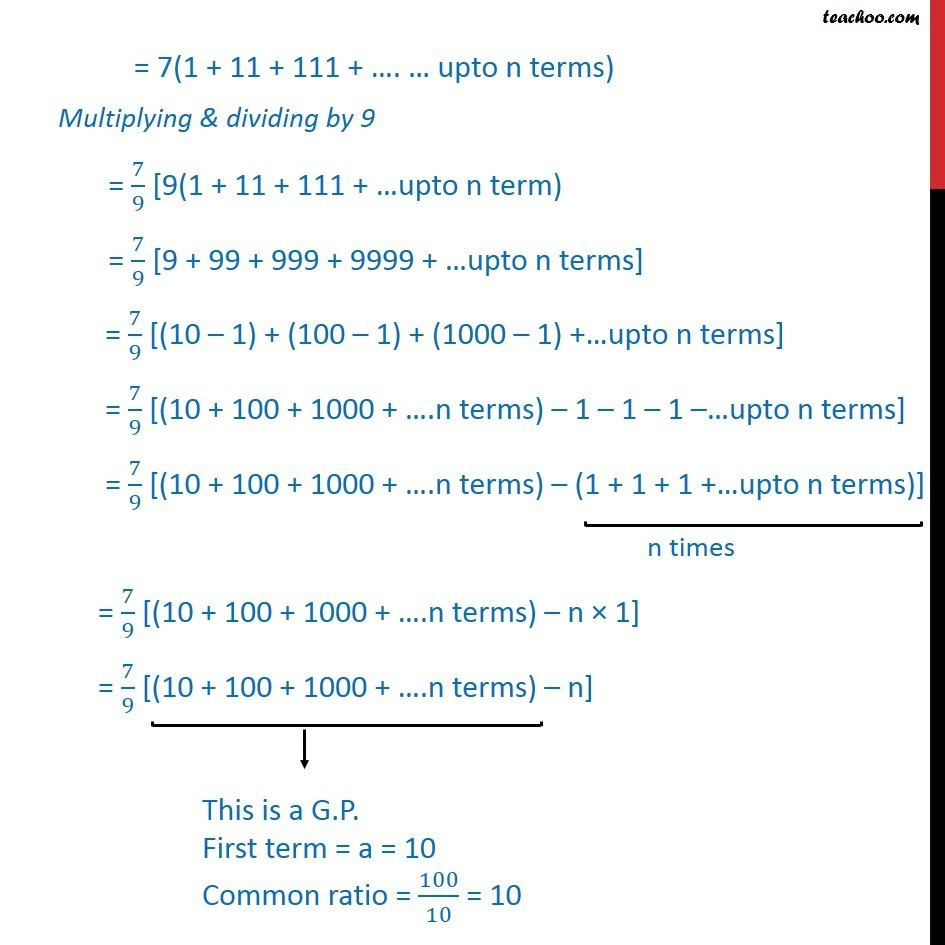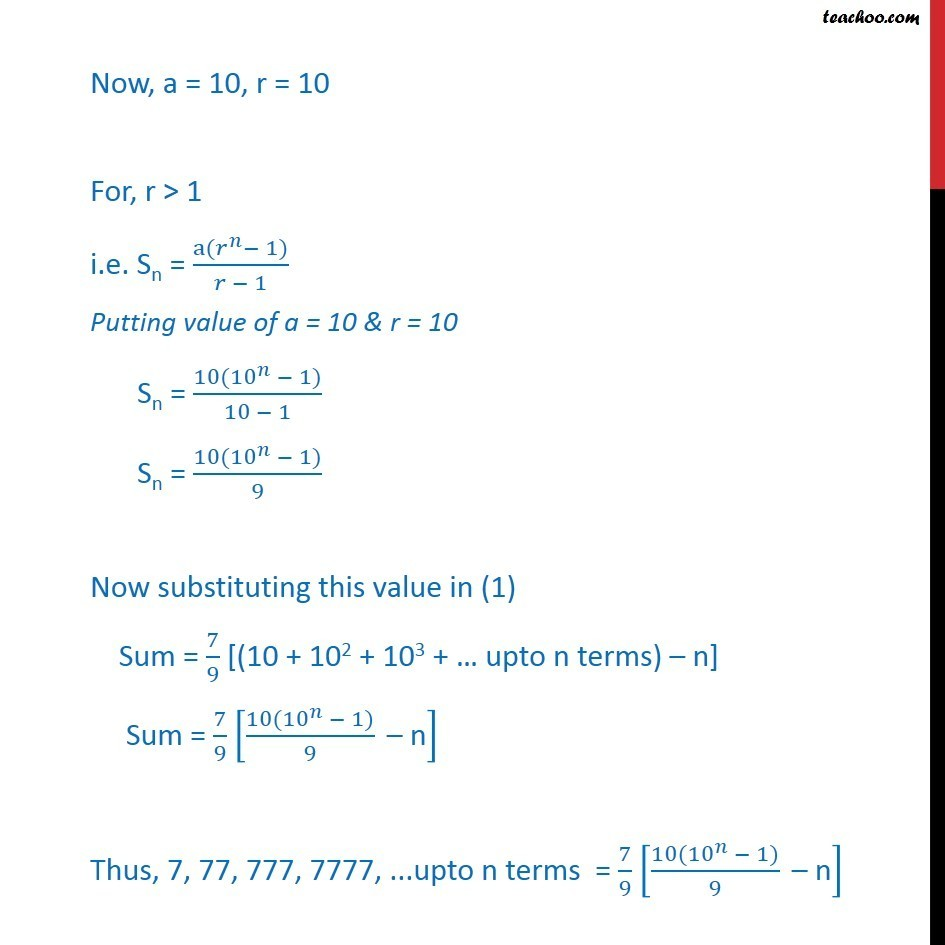Geometric Progression(GP): Formulae based

Chapter 8 Class 11 Sequences and Series
Concept wiseLearn in your speed, with individual attention - Teachoo Maths 1-on-1 Class

### Transcript

Example 10 Find the sum of the sequence 7, 77, 777, 7777, ... to n terms. 7, 77, 777, 7777, ... n terms Here, 77/7 = 11 & 777/77 = 10.09 Thus, ( )/( ) ( )/( ) i.e. common ratio is not same This is not a GP We need to find sum Sum = 7 + 77 + 777 + 7777 + ...upto n terms = 7(1 + 11 + 111 + . upto n terms) = 7(1 + 11 + 111 + . upto n terms) Multiplying & dividing by 9 = 7/9 [9(1 + 11 + 111 + upto n term) = 7/9 [9 + 99 + 999 + 9999 + upto n terms] = 7/9 [(10 1) + (100 1) + (1000 1) + upto n terms] = 7/9 [(10 + 100 + 1000 + .n terms) 1 1 1 upto n terms] = 7/9 [(10 + 100 + 1000 + .n terms) (1 + 1 + 1 + upto n terms)] = 7/9 [(10 + 100 + 1000 + .n terms) n 1] = 7/9 [(10 + 100 + 1000 + .n terms) n] Now, a = 10, r = 10 For, r > 1 i.e. Sn = (a( ^ 1))/( 1) Putting value of a = 10 & r = 10 Sn = (10( 10 ^ 1))/(10 1) Sn = (10( 10 ^ 1))/9 Now substituting this value in (1) Sum = 7/9 [(10 + 102 + 103 + upto n terms) n] Sum = 7/9 [(10( 10 ^ 1))/9 " n" ] Thus, 7, 77, 777, 7777, ...upto n terms = 7/9 [(10( 10 ^ 1))/9 " n" ]